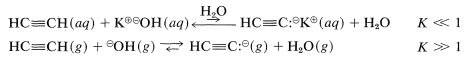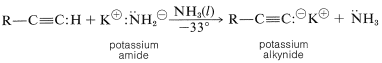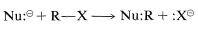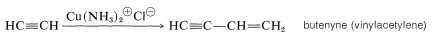# 11.8: Terminal Alkynes as Acids

$$\newcommand{\vecs}{\overset { \rightharpoonup} {\mathbf{#1}} }$$ $$\newcommand{\vecd}{\overset{-\!-\!\rightharpoonup}{\vphantom{a}\smash {#1}}}$$$$\newcommand{\id}{\mathrm{id}}$$ $$\newcommand{\Span}{\mathrm{span}}$$ $$\newcommand{\kernel}{\mathrm{null}\,}$$ $$\newcommand{\range}{\mathrm{range}\,}$$ $$\newcommand{\RealPart}{\mathrm{Re}}$$ $$\newcommand{\ImaginaryPart}{\mathrm{Im}}$$ $$\newcommand{\Argument}{\mathrm{Arg}}$$ $$\newcommand{\norm}{\| #1 \|}$$ $$\newcommand{\inner}{\langle #1, #2 \rangle}$$ $$\newcommand{\Span}{\mathrm{span}}$$ $$\newcommand{\id}{\mathrm{id}}$$ $$\newcommand{\Span}{\mathrm{span}}$$ $$\newcommand{\kernel}{\mathrm{null}\,}$$ $$\newcommand{\range}{\mathrm{range}\,}$$ $$\newcommand{\RealPart}{\mathrm{Re}}$$ $$\newcommand{\ImaginaryPart}{\mathrm{Im}}$$ $$\newcommand{\Argument}{\mathrm{Arg}}$$ $$\newcommand{\norm}{\| #1 \|}$$ $$\newcommand{\inner}{\langle #1, #2 \rangle}$$ $$\newcommand{\Span}{\mathrm{span}}$$$$\newcommand{\AA}{\unicode[.8,0]{x212B}}$$

A characteristic and synthetically important reaction of ethyne and 1-alkynes is salt (“acetylide”) formation with very strong bases. In such reactions the alkynes behaves as an acids in the sense that they give up protons to suitably strong bases:Water is too weak a base to accept protons from alkynes; consequently no measurable concentration of $$\ce{H_3O}^\oplus$$ is expected from the ionization of alkynes in dilute aqueous solutions. Therefore we have no quantitative measure of 1-alkyne acidity in aqueous solution other than that it probably is about $$10^{10}$$ times less acidic than water, as judged from measurements in other solvents to be discussed shortly. In the gas phase, however, the situation is reversed, and ethyne is a stronger acid than water:This reversal is of little practical value because organic reactions involving ions normally are not carried out in the gas phase. However, it should alert us to the tremendous role that solvents play in determining acidities by their abilities (some much more than others) to stabilize ions by the property known as solvation. (Section 11-8A.)

Liquid ammonia is a more useful solvent than water for the preparation of 1-alkyne salts. A substantial amount of the alkyne can be converted to the conjugate base by amide anions (potassium or sodium amide) because a 1-alkyne is a stronger acid than ammonia.The acidity of the terminal hydrogen in 1-alkynes provides a simple and useful test for 1-alkynes. With silver-ammonia solution ($$\ce{AgNO_3}$$ in aqueous ammonia), 1-alkynes give insoluble silver salts, whereas disubstituted alkynes do not:The silver “acetylides” appear to have substantially covalent carbon-metal bonds and are less ionic than sodium and potassium alkynides. Silver-ammonia solution may be used to precipitate 1-alkynes from mixtures with other hydrocarbons. The 1-alkynes are regenerated easily from the silver precipitates by treatment with strong inorganic acids. It should be noted, however, that silver alkynides may be shock sensitive and can decompose explosively, especially when dry.

## Thermodynamics of Solvation of Ions and Its Importance

Some idea of the importance of solvation can be gained from the calculated $$\Delta H$$ for the following process:

$\ce{Na^+ (g) + Cl^- (g) \rightarrow Na^+ (aq) + Cl^- (aq)}$

with $$Delta H^o = -187\,kcal$$.

The solvation energies of ions are so large that relatively small differences for different ions can have a very large effect on equilibrium constants. Thus, the ratio between the relative acidities of ethyne and water in the gas phase and in water of $$10^{12}$$ corresponds at $$25^\text{o}$$ to an overall $$\Delta G^0$$ difference in solvation energies of approximately $$16 \: \text{kcal}$$, which is less than $$10\%$$ of the total solvation energies of the ions. Further difficulties arise because of differences between solvation energies and interactions between the ions in different solvents. Thus the acidities of 1-alkynes relative to other acids have been found to change by a factor of $$10^{11}$$ in different solvents. For this reason, we must be particularly careful in comparing the rates and equilibrium constants of ionic reactions to take proper account of solvation and ion interaction effects.

An excellent rule of thumb is that, other things being equal, large ions are more stable than small ions in the gas phase, with the opposite being true in polar solvents, where small ions are more strongly solvated (thus more stable) than large ions. For comparison,From (1) minus (3), the solvation energy of gaseous $$\ce{Li}^\oplus$$ is $$47 \: \text{kcal mol}^{-1}$$ greater than $$\ce{K}^\oplus$$; and from (1) minus (2), that of $$\ce{F}^\ominus$$ is $$35 \: \text{kcal mol}^{-1}$$ greater than that of $$\ce{I}^\ominus$$. Such differences in solvation energies can have considerable effects on reactivity, and you may remember from Section 8-7E that $$\ce{F}^\ominus$$ is a weaker nucleophile than $$\ce{I}^\ominus$$, largely because of its greater solvation energy.

## Why is Ethyne a Stronger Acid than Ethane or Ethene?

If we compare acid strengths of the simple hydrocarbons, we find that ethyne is substantially more acidic than ethene or ethane in the gas phase or in solution. Why is this? The simplest explanation is that there is a direct connection between $$\ce{C-H}$$ acidity and the amount of $$s$$ character associated with the $$\sigma$$-bonding carbon orbital. Other things being equal, acidity increases with increasing $$s$$ character in the carbon orbital.Figure 11-4).Figure 11-4: Generalized valence bond (GVB) orbitals for one hydrogen of ethyne (left) and of ethane (right); see Section 6-7. The hydrogen and carbon nuclei are located in the $$xy$$ plane of the coordinate system at the positions indicated by crosses, the hydrogen nucleus being on the left. The long dashes indicate locations of change in orbital phase. The dotted lines are contour lines of electron amplitude of opposite phase to the solid lines. Notice how the contours of the ethyne hydrogen orbital are distorted toward carbon compared to those of the ethane hydrogen orbital. (Drawing courtesy W. A. Goddard III.)

## Alkynide Anions as Nucleophiles

1-Alkynes are very weak acids, hence their conjugate bases, $$\ce{RC \equiv C}^\ominus$$, are quite strong bases. These anions also are reactive carbon nucleophiles, and it is this property that makes them useful for organic synthesis. Recall from Chapter 8 that one of the most generally useful organic reactions is a displacement reaction in which an anionic nucleophile, $$\ce{Nu}^\ominus$$, attacks an alkyl derivative, $$\ce{RX}$$, to displace $$\ce{X}^\ominus$$ and form a new bond between carbon and the nucleophile:The displaced group $$\ce{X}$$ often is a halide ion (chloride, bromide, or iodide), and if the entering nucleophile $$\ce{Nu}^\ominus$$ is an alkynide anion, the reaction leads to formation of a carbon-carbon bond:With those $$\ce{RX}$$ derivatives that undergo nucleophilic displacement readily, this is a general method of forming a $$\ce{C-C}$$ bond, thereby leading to substituted alkynes. The alkynide salts generally used are those of lithium, sodium, potassium, or magnesium.

## Coupling Reactions of Alkynes

Another reaction of 1-alkynes that extends the carbon chain is a coupling reaction in which the alkyne dimerizes under the influence of a cuprous salt, usually cuprous ammonium chloride:This addition of one molecule of alkyne to another is formally analogous to the dimerization of alkenes under the influence of sulfuric acid (see Section 10-9), but the mechanisms are quite different.

If the reaction is carried out in the presence of an oxidizing agent, such as $$\ce{O_2}$$ or a cupric salt dissolved in pyridine (a weak base), a different product is obtained. Under these conditions, oxidative coupling occurs to give a conjugated diyne:Although the details of the mechanisms of these alkyne reactions are not known, it is likely that the ability of 1-alkynes to form carbon-metal bonds with metals such as copper is a key factor. Other oxidative coupling reactions occur with transition metals, and this will be discussed in detail in Chapter 31.